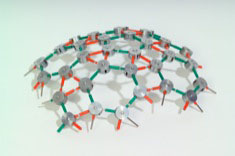# Self–assembling model of the protein shell of a spherical virus particleThe model illustrates the two main principles of the structure:Each unit represents a protein molecule and the red and green spokes represent the bonds or contacts between neighbouring molecules.

1. Self-assembly, in the sense that the model is built automatically out of the units, by following a simple rule, namely, join red to red bonds and green to green; and
2. The notion of a built-in curvature, which determines the size of the shell. In this particular model the curvature per unit is 4° (the angular departure from the level), so that there are 180 units in the complete structure. (The curvature of a complete sphere is 720°. Hence there are 720°/4°=180 units in the structure.)

The model has icosahedral symmetry being composed of hexagons and pentagons like a modern football. There are 12 pentagons (an essential part of the design) and there are 20 hexagons in this case.

## Mathematical note

The largest number of units that can be assembled into a mathematically perfect shell, where all the units are equivalent, that is both identical and identically connected to their neighbours, is 60. In the above model, the 180 units are said to be quasi-equivalent because they allow small variations in their bond distances and bond angles, allowing the formation of a multiple of 60 units, (reference DLD Caspar and A Klug, Cold Spring Harbour Symposium 27, 1-24, 1962). The particular design of the model is T=3 in the Caspar and Klug classification.